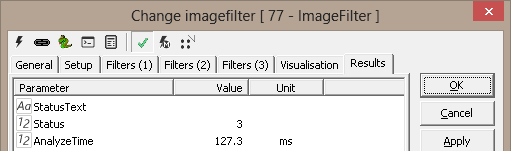Up | Logic - 1 | Blob1 - 61 | Intensity - 75 | PythonScript - 3 | BaseTool - 163 | SimpleMath - 21 | DummyTool - 20 | Goto - 59 | ColorMatcher - 54 | ImageCombiner - 74 | ImageFilter - 77 | ImageConverter - 55 | BarCodeReader - 56 | EasyBarCode - 72 | EasyMatrixCode - 73 | CommitImage - 159 | Separator - 102 Release Notes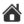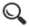ImageFilter - 77
The ImageFilter tool has the following filter possibilities
• Trend removal
• Median Filter
• FIR Filter
• Lowpass/highpass filter
• Sobel filter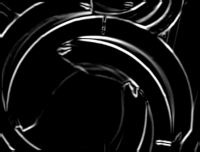The image processed with a sobel filter.

Demonstration Profile

Setup

Reference - Reference system selection

ROI - image for calculation and ROI (region of interest)

• Whole image - ignore ROI settings and use all of the image
• Center-X - x
• Center-Y - y
• dX - height
• dY - width

The ROI is managed by the buttons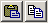• Paste - paste the ROI from the image to the scorpion clipboard
• Copy - copy the ROI to the image from the scorpion clipboard

Point & Click Clipboard Support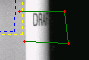The rectangular ROI is defined by four points.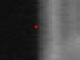One point will change the center point.

More on Image Operations.

Output Image

• Output to image - name of image to present result
• Pixel size (pitch) - correspondence between input reference units and output image pixels. A value of 1 means one output pixel per input unit, 2 means one output pixel per two input units.
• Resulting image in pixels - the actual image size is shown

Filter Order - selects the active filters and set the executing order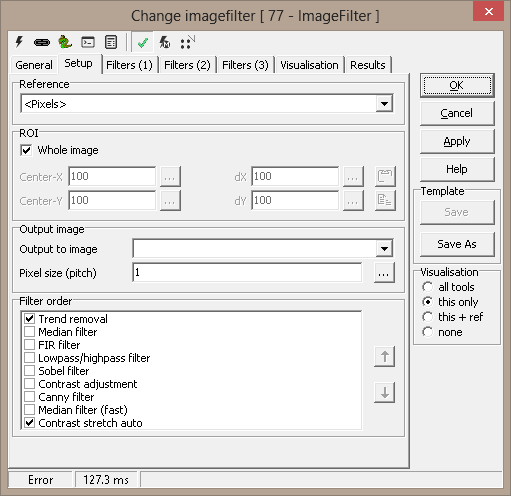Filters (1)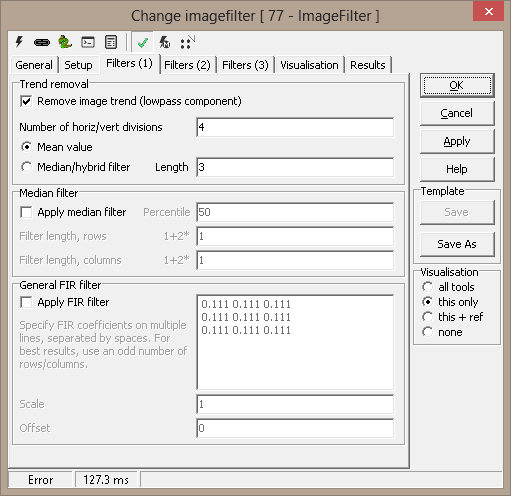Trend removal

The image trend is the overall, or background, intensity variations. The image is subdivided into rectangles, and each rectangle is analysed, attempting to extract the background. Two methods can be used:

• Mean value - simple, but not always effective
• Median/hybrid - the mean value of the Length middle pixel values

The extracted values are used to fit a plane of grayscale values over the image; by adjusting each pixel by the plane's height at its position, the image is effectively evened out (1st order only).

Median filter

This is a simple percentile median done with a search window given by the length in rows and columns. To guarantee an odd-numbered filter size (in x and y), the input number is modified as specified. A value of 0 means filterlength of 1, a value of 1 means filterlength 3 etc. The Percentile value specifies which of the sorted values to output.

General FIR filter

You can type/paste filter coefficients in the edit rectangle. The resulting filter size will be the number of rows times the largest number of coefficients per line. To avoid shifts in the image, an odd number of rows and columns must be used, as well as a symmetrical filter matrix (in both x and y).

Scale and offset are multiplied/added after the FIR filter.

Filters (2)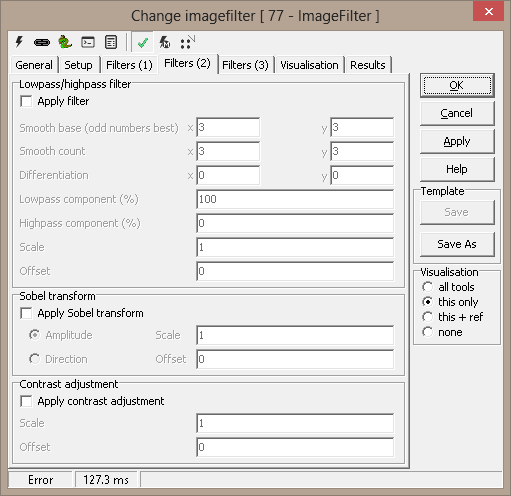Lowpass/highpass filter

This is a simple square lowpass FIR filter with equal coefficients. The filter can be applied several times, and the result can be differentiated in x or y direction (or both). To synthesise a highpass filter, lowpass values are simply subtracted from the original values. NOTE: for this to be possible, an odd number of coefficients ("smooth base") must be specified. The lowpass and highpass results can then be combined by a percentage.

Scale and offset are multiplied/added after the filter.

Sobel transform

The Sobel transform calculates gradients in the picture. For most applications, the amplitude is used as the result, but the angle (or direction) can also be used. Here, he circle (0-360 degrees) is represented by the grayscale values 0-255.

This is simply a multiply/add for all pixel values.

Filters (3)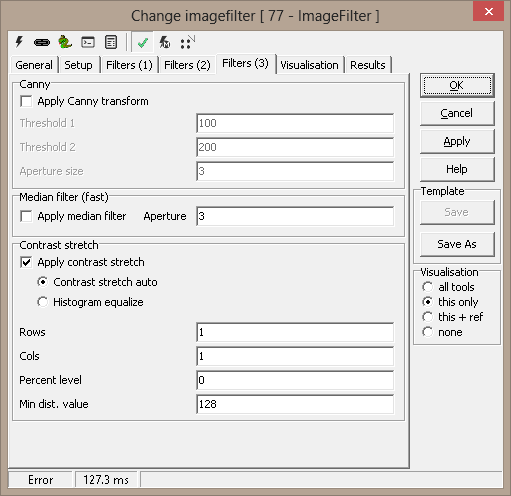Visualisation

 ROI Region of interest

Results